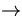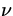# Nuclear Reactions

The reactions considered so far occur spontaneously, and involve a conversion of mass into (mainly) kinetic energy of the decay products. There is another class of nuclear reactions which can be induced, an example of which is bombarding a nitrogen nucleus with an alpha particle:

 42 He + 147 N178 O + 11 H . (15)

In this case the sum of the masses of the elements on the left-hand-side of this equation is less than the sum of the masses of the decay products on the right-hand-side. In order for the reaction to proceed some initial kinetic energy must be supplied to the left-hand-side reactants; such energy, again through Einstein's relation of Eq. (29.2), gets converted into mass of the products. Some examples will illustrate this later. There are two general types of such nuclear reactions:
• fusion, where lighter elements fuse'' into heavier ones. An example of this is the following reaction:

 11 H + 11 H21 H + 01 e +. (16)

Fusion reactions are the main source of energy for the sun, and are also used in certain types of nuclear weapons.
• fission, where heavier elements are broken into lighter ones. An example of this is the following reaction:

 10 n + 23592 U14156 Ba + 9236 Kr + 3 10 n . (17)

An interesting feature of this neutron-induced reaction is the presence of neutrons in the products; it turns out that the initial kinetic energy of the reacting neutrons can be relatively small, and the released neutrons can induce reactions in nearby Uranium atoms. Such a chain reaction has important consequences in the design of nuclear reactors, as well as nuclear weapons.
As we will see later in some examples, the typical energies involved in nuclear reactions are of the order of MeV ( 106 eV), compared to the eV scale of chemical reactions.

Due to the relatively large kinetic energy needed for the initial reactants, fusion-based nuclear reactors are not as of yet economically feasible. However, given the risks of runaway chain reactions, as well as the presence of dangerous, long-lived radioactive reactants and products in fission reactors, much research is being invested into this form of energy production.Next: Problems Up: Nuclear Physics Previous: Measuring Radioactivity